• 思考:气温发生了怎样的变化？ 在哪段时间气温升高，在哪段气温降低？
• 1.判断具体函数单调性的方法对于给出具体解析式的函数，由函数单调性的定义出发，本文列举的判断函数单调性的方法有如下几种：1.1 定义法单调函数的定义：一般地，设f(x)为定义在D上的函数。若对任何x1、x2∈D，当x1...

掌握函数单调性的判定方法是学好高中数学必不可少的一个重要的知识点。
1.判断具体函数单调性的方法
对于给出具体解析式的函数，由函数单调性的定义出发，本文列举的判断函数单调性的方法有如下几种：
1.1 定义法
单调函数的定义：一般地，设f(x)为定义在D上的函数。若对任何x1、x2∈D，当x1
(1)f(x1)
(2)f(x1)>f(x2)，则称f(x)为D上的减函数。
用单调性的定义判断函数单调性的方法叫定义法。
利用定义来证明函数y=f(x)在给定区间D上的单调性的一般步骤：
(1)设元，任取x1、x2∈D,且x1
(2)作差f(x1)-f(x2);
(3)变形(普遍是因式分解和配方);
(4)断号(即判断f(x1)-f(x2)与0的大小);
(5)定论(即指出函数f(x)在给定的区间D上的单调性)。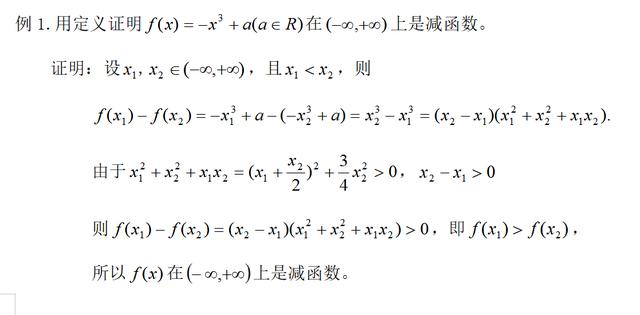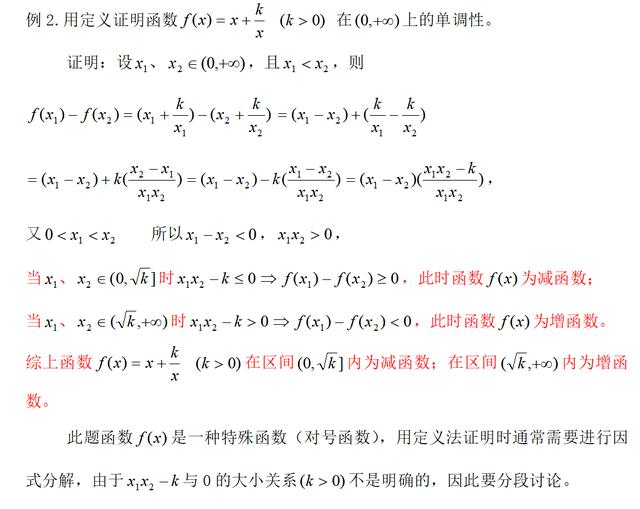在解决问题时，定义法是最直接的方法，也是我们首先考虑的方法，虽说这种方法思路比较清晰，但通常过程比较繁琐。
1.2 函数性质法
函数性质法是用单调函数的性质来判断函数单调性的方法。函数性质法通常与我们常见的简单函数的单调性结合起来使用。对于一些常见的简单函数的单调性如下图：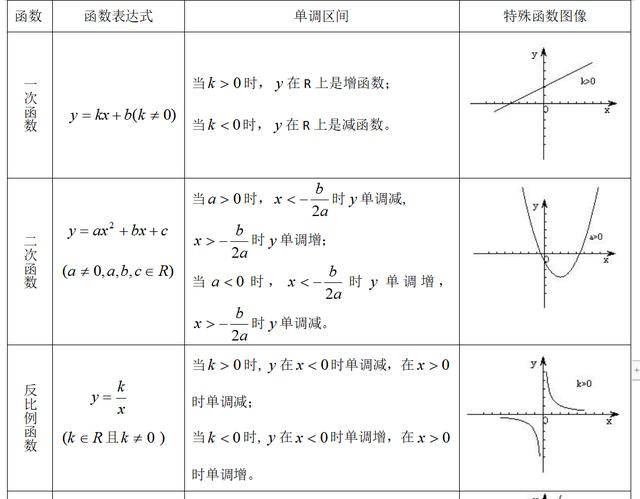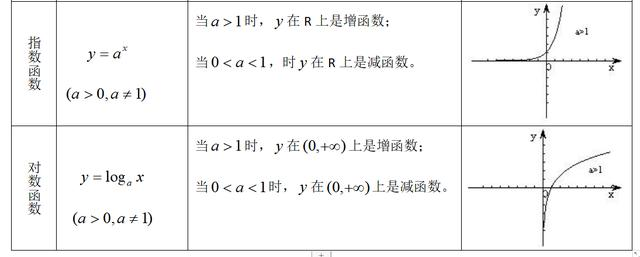对于一些常用的关于函数单调的性质可总结如下一些结论：
⑴.f(x)与f(x)+C单调性相同。(C为常数)
⑵.当k>0时，f(x)与kf(x)具有相同的单调性;当k<0时， f(x)与kf(x)具有相反的单调性。
⑶.当f(x)恒不等于零时，f(x)与1/f(x)具有相反的单调性。
⑷.当f(x)、g(x)在D上都是增(减)函数时，则f(x)+g(x)在D上是增(减)函数。
⑸.当f(x)、g(x)在D上都是增(减)函数且两者都恒大于0时，f(x)g(x)在D上是增(减)函数;当f(x)、g(x)在D上都是增(减)函数且两者都恒小于0时，f(x)g(x)在D上是减(增)函数。
⑹.设y=f(x),x∈D为严格增(减)函数，则f(x)必有反函数，且反函数在其定义域f(D)上也是严格增(减)函数。
我们可以借助以上简单函数的单调性来判断函数的单调性，下面我们来看以下几个例子：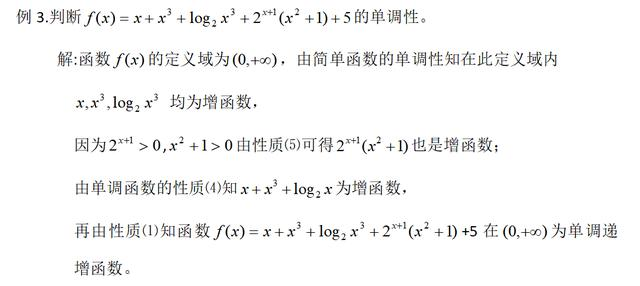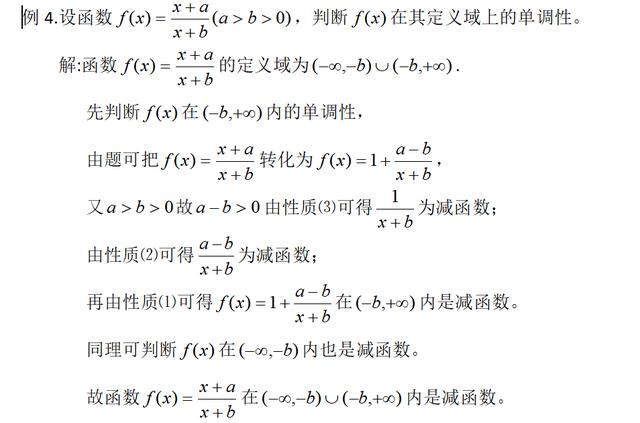函数性质法只能借助于我们熟悉的单调函数去判断一些函数的单调性，因此首先把函数等价地转化成我们熟悉的单调函数的四则混合运算的形式，然后利用函数单调性的性质去判断，但有些函数不能化成简单单调函数四则混合运算形式就不能采用这种方法。
1.3 图像法
用函数图像来判断函数单调性的方法叫图像法。根据单调函数的图像特征，若函数f(x)的图像在区间D上从左往右逐渐上升则函数f(x)在区间D上是增函数;若函数f(x)图像在区间D上从左往右逐渐下降则函数f(x)在区间D上是减函数。
例5. 如图是定义在闭区间[-5,5]上的函数的图像，试判断其单调性。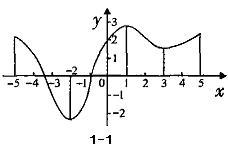解：由图像可知：函数y=f(x)的单调区间有[-5,-2)，[-2,1)，[1,3)，[3,5)。
其中函数y=f(x)在区间[-5,-2)，[1,3)上的图像是从左往右逐渐下降的，则函数y=f(x)在区间[-5,-2)，[1,3)为减函数;函数y=f(x)在区间[-2,1)，[3,5]上的图像是从往右逐渐上升的，则函数y=f(x)在区间[-2,1)，[3,5]上是增函数。
例6.利用函数图像判断函数f(x)=x+1;‚g(x)=2x;ƒh(x)=2x+x+1在[-3,3]上的单调性。
分析：观察三个函数，易见h(x)=f(x)+g(x)，作图一般步骤为列表、描点、作图。首先作出f(x)=x+1和g(x)=2x的图像，再利用物理学上波的叠加就可以大致作出h(x)=2x+x+1的图像，最后利用图像判断函数h(x)=2x+x+1的单调性。
解:作图像如下所示：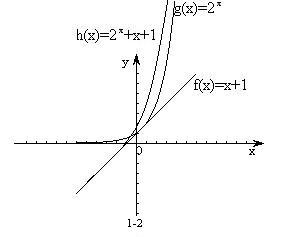由以上函数图像得知函数f(x)=x+1在闭区间[-3,3]上是单调增函数;
g(x)=2x在闭区间[-3,3]上是单调增函数;
利用物理上波的叠加可以直接大致作出ƒh(x)=2x+x+1在闭区间[-3,3]上图像，即ƒh(x)=2x+x+1在闭区间[-3,3]上是单调增函数。
事实上本题中的三个函数也可以直接用函数性质法判断其单调性。
用函数图像法判断函数单调性比较直观，函数图像能够形象的表示出随着自变量的增加，相应的函数值的变化趋势，但作图通常较烦。对于较容易作出图像的函数用图像法比较简单直观，可以类似物理上波的叠加来大致画出图像。而对于不易作图的函数就不太适用了。但如果我们借助于相关的数学软件去作函数的图像，那么用图像法判断函数单调性是非常简单方便的。
1.4 复合函数单调性判断法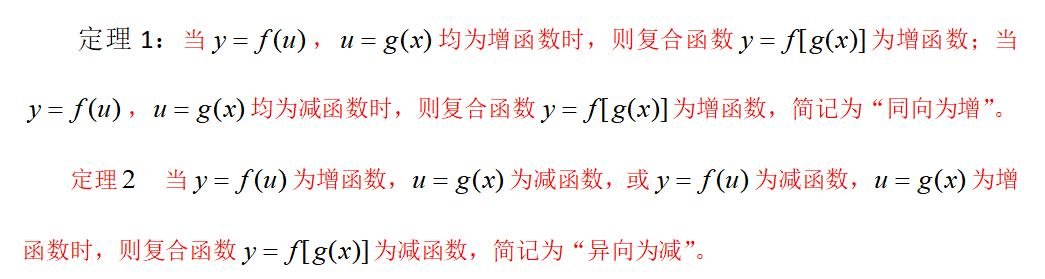归纳此定理，可得口诀：同增异减。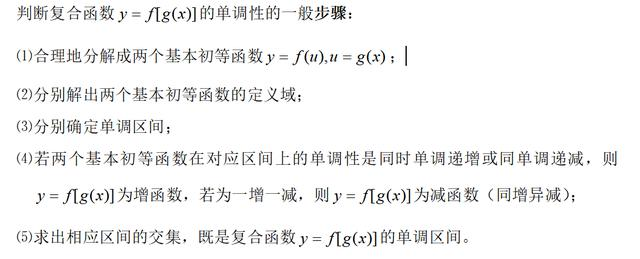以上步骤可以用八个字简记“一分”，“二求”，“三定”，“四交”。利用“八字”求法可以解决一些复合函数的单调性问题。下面我们就用“八字”求法来判断函数的单调性。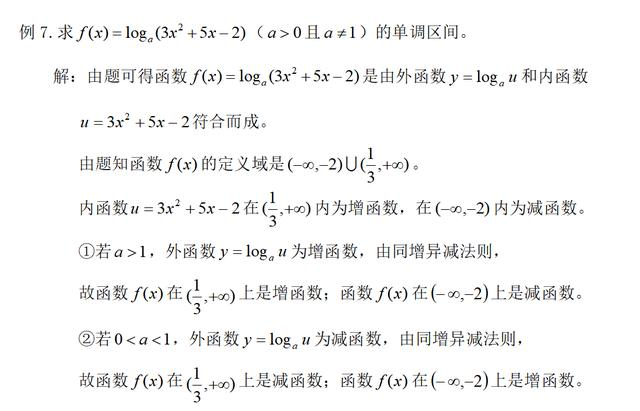1.5 导数法
我们在前面也曾利用函数图像的特点判断函数的增减性，图像上升则递增，图像下降则递减.用定义法、图像法等这些初等方法来判断函数的单调性，一般比较繁杂，下面我们将以导数为工具来判断函数的单调性。函数f(x)的导数反映了函数增加或减小的快慢，即变化率.因此我们可以利用导数判断函数的单调性.这种用导数的符号来判断函数单调性的方法叫导数法。在给定区间内只要能求出其导数我们就可以用导数法来判断函数单调性。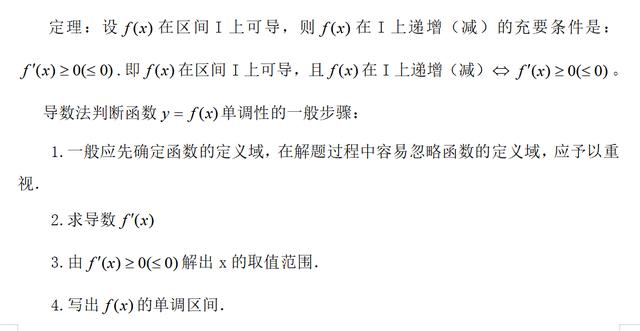下面我们来看下面几个例题：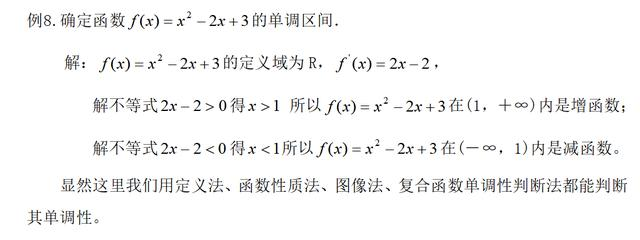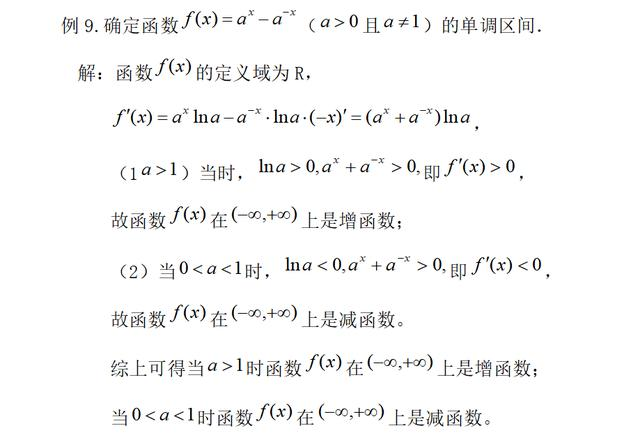2.判断抽象函数单调性的方法
如果一个函数没有给出具体解析式，那么这样的的函数叫做抽象函数。抽象函数没有具体的解析式，需充分提取题目条件给出的信息。
2.1 定义法
通过作差(或者作商)，根据题目提出的信息进行变形，然后与0(或者1)比较大小关系来判断其函数单调性。通常有以下几种方法：
2.1.1 凑差法
根据单调函数的定义，设法从题目中“凑出”“f(x1)-f(x2)”的形式，然后比较f(x1)-f(x2)与0的大小关系。
例11.已知函数f(x)对任意实数m、n均有f(m+n)=f(m)+f(n)，且当m>0时，
f(m)>0，试讨论函数f(x)的单调性。
解：由题得f(m+n)-f(m)=f(n)，
令x1=m+n,x2=m，且x1>x2，则n=x1-x2>0
又由题意当m>0时，f(m)>0,得
f(x1)-f(x2)=f(m+n)-f(m)=f(n)>0，
所以函数f(x)为增函数。
2.1.2添项法
弄清题目中的结构特点，采用加减添项或乘除添项，以达到能判断“f(x1)-f(x2)”与0大小关系的目的。
例12.(同例11)已知函数f(x)对任意实数m、n均有f(m+n)=f(m)+f(n)，且当m>0时，f(m)>0，试讨论函数f(x)的单调性。
解:任取x1、x2 ∈R，x10,
f(x2)-f(x1)=f[(x2-x1)+x1]-f(x1)
由题意函数f(x)对任意实数m、n均有f(m+n)=f(m)+f(n)
且当m>0时，f(m)>0,得
f(x2)-f(x1)=f(x2-x1)>0，
所以函数f(x)为增函数。
2.1.3 增量法
由单调性的定义出发，任取x1、x2 ∈R，x10),然后联系题目提取的信息给出解答。
例13.已知函数f(x)对任意实数m、n均有f(m+n)=f(m)+f(n)，且当m>0时，f(m)>0，试讨论函数f(x)的单调性。
解：任取x1、x2 ∈R，x10)
由题意函数f(x)对任意实数m、n均有f(m+n)=f(m)+f(n)，得
f(x2)-f(x1)=f(x1+γ)-f(x1)=f(γ)
又由当m>0时，f(m)>0得
f(x2)-f(x1)=f(γ)>0
所以函数f(x)为增函数。
2.1.4 放缩法
利用放缩法，判断f(x1)与f(x2)的大小关系，从而得f(x)在其定义域内的单调性。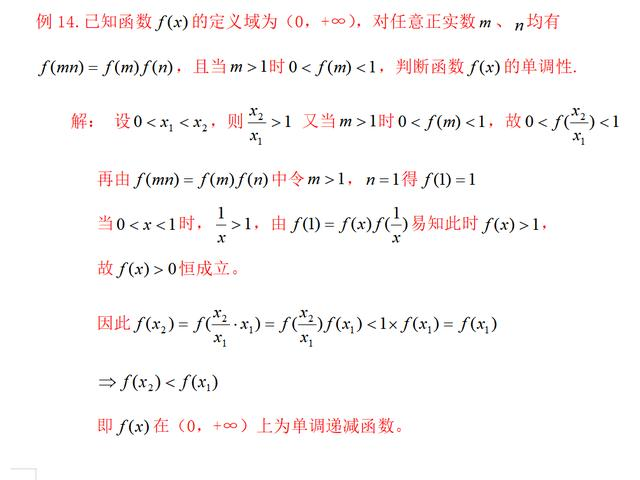对于抽象函数，由于抽象函数没有具体的解析式，因此需充分提取题目条件给出的信息,观察结构特点。用定义法判定抽象函数单调性比较适用于那种对于定义域内任意两个数x1、x2 ，当x1
总结：函数单调性是函数的一个非常重要的性质，本文从单调性的定义入手，总结了判断单调性的常见方法。本文把函数分为具体函数和抽象函数两大类进行讨论，对于每类函数都给出了判定单调性的若干方法。对于具体的函数，我们可以用多种方法去判断其单调性，特别地导数法是普遍适用的，若借助于计算机，那么图像法也是最简单最直观的。对于抽象函数的单调性问题，我们给出了用定义法及列表法。这种题型不仅抽象，而且综合性较强，对学生的思维能力有很高的要求，学生往往很难发现数学符号与数学语言之间的内在关系。因此在判断函数单调性的问题上，应灵活选择恰当的方法，从而使解题过程最简单。
注意：文中讲的是函数单调性的判断方法，要注意区分函数单调性的证明与判断的不同。函数单调性的证明只能用定义法和导数法，而函数单调性的判断除定义法和导数法，还可以使用文中介绍的各种方法进行判断。展开全文•文档
•文档
•文档
•文档
•文档
•文档
•文档
•文档
•文档
• 函数单调性定义 函数值随着自变量在定义域内的增大而增大，则函数在该区间内单调递增； 函数值随着自变量在定义域内的增大而减小，则函数在该区间内单调递减。 应用 判断实数根的个数。 步骤 1、对方程...
函数单调性定义
    函数值随着自变量在定义域内的增大而增大，则函数在该区间内单调递增；
函数值随着自变量在定义域内的增大而减小，则函数在该区间内单调递减。
应用
    判断实数根的个数。
步骤
1、对方程移项，使得方程一侧等于0，并令f(x)=方程的另一侧。
2、若f(x)可导，则令f(x)=0，求出x；
3、将求出的x带入到f(x)中，求出函数值。
4、根据刚才求出的x，分别判断f(x)在一定区间内的正负号；
5、根据第3、4条得出结论。
例题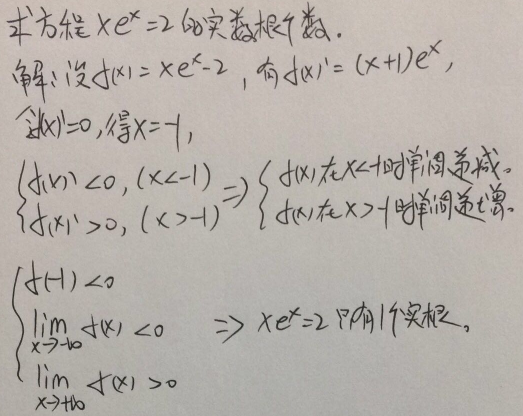展开全文• 本文介绍了利用导数判断函数单调性、凹凸性、极值相关的概念和定理，通过本文的介绍，可以熟悉通过导数判断函数单调性、凹凸性、极值以及求最值的原理和方法。最后，通过一阶导数和二阶导数确定了函数的单调性、凹凸...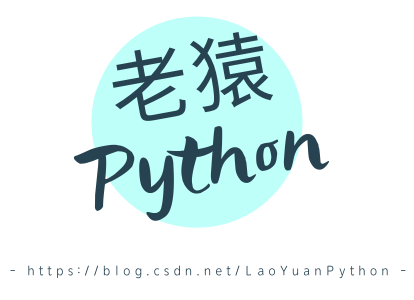一、单调性判断定理
定理： 设函数y=f(x)在[a，b]上连续，在(a，b)内可导。 (1)如果在(a，b)内f(x)≥0，且等号仅在有限多个点处成立，那么函数y=f(x)在[a，b]上单调增加; (2)如果在(a，b)内f(x)≤0，且等号仅在有限多个点处成立，那么函数y=f(x)在[a，b]上单调减少。
证明思路： 利用拉格朗日中值公式，可以正得任意两点的函数值差等于某点导数与两点x值的差的乘积，因此x值的差决定了函数值的差的符号。
另外对于导数为0的点，将区间分成了2部分，每部分的单调性跟随导数的值与自变量的差的值，这表明两个区间的单调性遵循定理的要求，则两个区间叠加后也会遵循。
二、曲线凹凸性判断
1、凹凸性的判断规则
函数曲线的上升或下降反映了函数的单调性，而曲线在上升或下降过程中，还存在一个弯曲方向的问题，如图：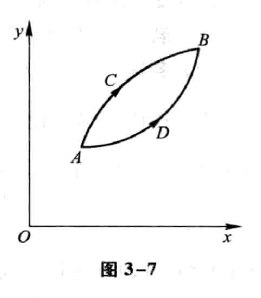曲线的凹凸性在几何图形上的描述：通过曲线上任取两点，如果连接这两点的直线（弦）总是位于这两点曲线弧的上方，则曲线是向下弯曲（凹），如果弦总是位于曲线弧的上方，则曲线是向上凸的。
曲线的凹凸性函数形式的表达： 设f(x)在区间I上连续，如果对I上任意两点x1、x2恒有：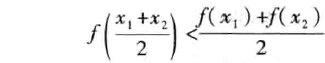那么称f(x)在I上的图形是(向上)凹的(或凹弧)，如果恒有：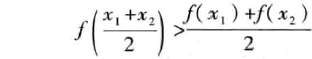那么称/(x)在I上的图形是(向上)凸的(或凸弧)。
2、曲线凹凸性判断定理
设f(x)在[a,b]上连续，在(a,b)内具有一阶和二阶导数，那么 (1)若在(a,b)内f”(x)>0,则f(x)在[a,b]上的图形是凹的; (2)若在(a,b)内f”(x)<0,则f(x)在[a,b]上的图形是凸的。
证明思路：
任取区间内两点x1、x2，假设x2>x1，然后取x0=(x1+x2)/2，记h=x0-x1=x2-x0，分别对区间[x1,x0]、[x0,x2]应用柯西中值定理，得到的两个式子相减后再应用柯西中值定理，如果函数f(x)的二阶导数大于0，就可以得到：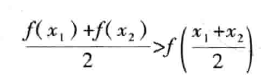三、极值
1、定义
设函数f(x)在点x0的某邻域U(x0)内有定义，如果对于去心邻域U°(x0)内的任一x，有 f(x)<f(x0)(或f(x)>f(x0))，那么就称f(x0)是函数f(x)的一个极大值(或极小值)。
备注：去心邻域实际的表示不是U°，而是在U上面一个小圈，但无法用文字输入，因此老猿所有的博文都用了U°来表示，实际的符号应该是：函数的极大值与极小值统称为函数的极值，使函数取得极值的点称为极值点。
函数的极大值和极小值概念是局部性的。如果f(x0)是函数f(x)的一个极大值，那是就x0附近的一个局部范围来说，f(x0)是f(x)的一个最大值，如果就f(x)的整个定义域来说f(x0)不见得是最大值。关于极小值也类似。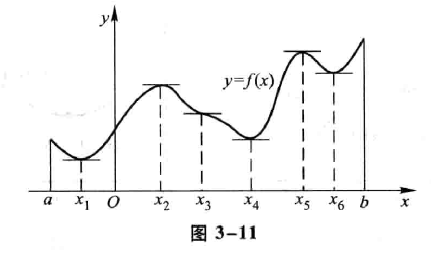在图3-11中，函数f(x)有两个极大值：f(x2)、f(x5)，三个极小值：f(x1)、f(x4)、f(x6)，其中极大值f(x2)比极小值f(x0)还小。就整个区间[a，b]来说，只有一个极小值f(x1)同时也是最小值，而没有一个极大值是最大值。
2、定理1(必要条件)
定理：设函数f(x)在x0处可导，且在x0处取得极值，则f’(x0)=0。
定理1就是说：可导函数f(x)的极值点必定是它的驻点，但反过来，函数的驻点却不一定是极值点。
例如，f(x)=x3的导数f’(x)=3x2，f’(0)=0，因此x=0是这可导函数的驻点，但x=0却不是这函数的极值点。
所以，函数的驻点只是可能的极值点。此外，函数在它的导数不存在的点处也可能取得极值。
3、定理2(第一充分条件)
定理：设函数f(x)在x0处连续，且在x0的某去心邻域U°(x0,δ)内可导。 (1)若x∈(x0-δ,x0)时，f’(x)>0，而x∈(x0,x0+δ)时，f’(x)<0，则f(x)在x0处取得极大值； (2)若x∈(x0-δ,x0)时，f’(x)<0，而x∈(x0,x0+δ)时，f’(x)>0，则f(x)在x0处取得极小值； (3)若x∈U°(x0,δ)时，f’(x)的符号保持不变，则f(x)在x0处没有极值。
定理2也可简单地这样说：当x在x0的邻近渐增地经过x0时，如果f’(x)的符号由正变负，那么f(x)在x0处取得极大值；如果f(x)的符号由负变正，那么f(x)在x0处取得极小值；如果f’(x)的符号并不改变，那么f(x)在x0处没有极值。
根据上面的两个定理，如果函数f(x)在所讨论的区间内连续，除个别点外处处可导，那么就可以按下列步骤来求f(x)在该区间内的极值点和相应的极值： (1)求出导数f’(x); (2)求出f(x)的全部驻点与不可导点; (3)考察f’(x)的符号在每个驻点或不可导点的左、右邻近的情形，以确定该点是否为极值点；如果是极值点，进一步确定是极大值点还是极小值点； (4)求出各极值点的函数值，就得函数(x)的全部极值。
4、定理3(第二充分条件)
定理：设函数f(x)在x0处具有二阶导数且f’(x0)=0，f"(x0)≠0，则 (1)当f"(x0)<0时，函数f(x)在x0处取得极大值; (2)当f”(x)>0时，函数f(x)在x0处取得极小值。
证明思路： 根据导数的定义有：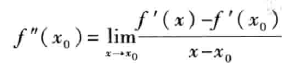而f’(x0)=0，可以得到x在x0的足够小的去心邻域时，上式右边不带极限符号的表达式的运算结果的符号取决于f"(x0)的符号，也可以得出f’(x)的符号与x-x0的符号的关系，再结合定理2就可以证明上述结论。
定理3表明：
如果函数f(x)在驻点x0处的一阶导数f’(x0)=0、二阶导数f”(x0)≠0，那么该驻点x0一定是极值点，并且可以按二阶导数f”(x0)的符号来判定f(x0)是极大值还是极小值。
但如果f"(x)=0，那么定理3就不能应用。事实上，当f’(x0)=0，f"(x)=0时(x)在x处可能有极大值，也可能有极小值，也可能没有极值。
例如，f(x)=-x4,f2(x)=x4,f3(x)=x3这三个函数在x=0处就分别属于这三种情况。
因此，如果函数在驻点处的二阶导数为零，那么可以用一阶导数在驻点左右邻近的符号来判定；如果函数在驻点处有f"(x0)=…=f(n-l)(x0)=0，f(n)(x0)≠0，那么也可利用具有佩亚诺余项的泰勒公式来讨论判定)。
四、求最值的方法
假定函数f(x)在闭区间[a，b]上连续，在开区间(a，b)内除有限个点外可导，且至多有有限个驻点。在上述条件下，我们来讨论f(x)在[a，b]上的最大值和最小值的求法。
首先，由闭区间上连续函数的性质可知，f(x)在[a，b]上的最大值和最小值一定存在。
其次，如果最大值(或最小值)f(x0)在开区间(a，b)内的点x0处取得，那么，按f(x)在开区间内除有限个点外可导且至多有有限个驻点的假定，可知f(x0)一定也是f(x)的极大值(或极小值)，从而x0一定是f(x)的驻点或不可导点。又f(x)的最大值和最小值也可能在区间的端点处取得。
因此，可用如下方法求f(x)在[a，b]上的最大值和最小值： (1)求出f(x)在(a，b)内的驻点及不可导点; (2)计算f(x)在上述驻点、不可导点处的函数值及f(a)、f(b); (3)比较(2)中诸值的大小，其中最大的便是f(x)在[a，b]上的最大值，最小的便是f(x)在[a，b]上的最小值。
在求函数的最大值(或最小值)时，特别值得指出的是下述情形：f(x)在一个区间(有限或无限，开或闭)内可导且只有一个驻点x0，并且这个驻点x0是函数f(x)的极值点，那么，当f(x0)是极大值时f(x0)就是f(x)在该区间上的最大值(图3-15(a))；当f(x0)是极小值时f(x0)就是f(x)在该区间上的最小值(图3-15(b))，在应用问题中往往遇到这样的情形。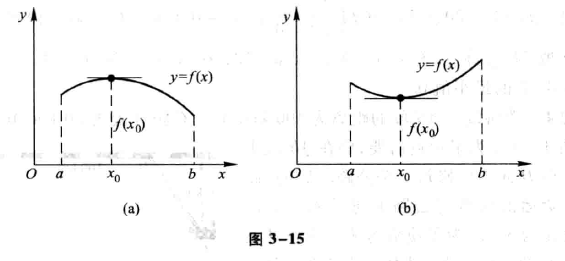五、借助导数描绘函数图形
借助于一阶导数的符号，可以确定函数图形在哪个区间上上升，在哪个区间上下降；
借助于二阶导数的符号，可以确定函数图形在哪个区间上为凹，在哪个区间上为凸，在什么地方有拐点。
知道了函数图形的升降、凹凸以及拐点后，也就可以掌握函数的形态，并把函数的图形画得比较准确。
利用导数描绘函数图形的一般步骤如下：
第一步 确定函数y=f(x)的定义域及函数所具有的某些特性(如奇偶性、周期性等)，并求出函数的一阶导数f’(x)和二阶导数f"(x)；第二步 求出一阶导数’(x)和二阶导数f”(x)在函数定义域内的全部零点，并求出函数f(x)的间断点及f’(x)和f”(x)不存在的点，用这些点把函数的定义域划分成几个部分区间；第三步 确定在这些部分区间内f’(x)和f“(x)的符号，并由此确定函数图形的升降、凹凸和拐点；第四步 确定函数图形的水平、铅直渐近线以及其他变化趋势；第五步 算出f’(x)和f”(x)的零点以及不存在的点所对应的函数值，定出图形上相应的点；为了把图形描绘得准确些，有时还需要补充一些点，然后结合第三、四步中得到的结果，联结这些点画出函数y=f(x)的图形。
现在，随着现代计算机技术的发展，借助于计算机和许多数学软件，可以方便地画出各种函数的图形。但是，如何识别机器作图中的误差，如何掌握图形上的关键点，如何选择作图的范围等，从而进行人工干预，仍然需要我们有运用微分学的方法描绘函数图形的基本知识。
六、小结
本文介绍了利用导数判断函数单调性、凹凸性、极值相关的概念和定理，通过本文的介绍，可以熟悉通过导数判断函数单调性、凹凸性、极值以及求最值的原理和方法。最后，通过一阶导数和二阶导数确定了函数的单调性、凹凸性、极值点之后，就可以描绘出函数的几何图形。
说明：
本文内容是老猿学习同济版高数的总结，有需要原教材电子版以及OpenCV、Python基础知识、、图像处理原理介绍相关电子资料，或对文章内有有疑问咨询的，请扫博客首页左边二维码加微信公号，根据加微信公号后的自动回复操作。
更多人工智能数学基础请参考专栏《人工智能数学基础》。
写博不易，敬请支持：
如果阅读本文于您有所获，敬请点赞、评论、收藏，谢谢大家的支持！
关于老猿的付费专栏
付费专栏《https://blog.csdn.net/laoyuanpython/category_9607725.html 使用PyQt开发图形界面Python应用》专门介绍基于Python的PyQt图形界面开发基础教程，对应文章目录为《 https://blog.csdn.net/LaoYuanPython/article/details/107580932 使用PyQt开发图形界面Python应用专栏目录》；付费专栏《https://blog.csdn.net/laoyuanpython/category_10232926.html moviepy音视频开发专栏 )详细介绍moviepy音视频剪辑合成处理的类相关方法及使用相关方法进行相关剪辑合成场景的处理，对应文章目录为《https://blog.csdn.net/LaoYuanPython/article/details/107574583 moviepy音视频开发专栏文章目录》；付费专栏《https://blog.csdn.net/laoyuanpython/category_10581071.html OpenCV-Python初学者疑难问题集》为《https://blog.csdn.net/laoyuanpython/category_9979286.html OpenCV-Python图形图像处理 》的伴生专栏，是笔者对OpenCV-Python图形图像处理学习中遇到的一些问题个人感悟的整合，相关资料基本上都是老猿反复研究的成果，有助于OpenCV-Python初学者比较深入地理解OpenCV，对应文章目录为《https://blog.csdn.net/LaoYuanPython/article/details/109713407 OpenCV-Python初学者疑难问题集专栏目录 》付费专栏《https://blog.csdn.net/laoyuanpython/category_10762553.html Python爬虫入门 》站在一个互联网前端开发小白的角度介绍爬虫开发应知应会内容，包括爬虫入门的基础知识，以及爬取CSDN文章信息、博主信息、给文章点赞、评论等实战内容。
前两个专栏都适合有一定Python基础但无相关知识的小白读者学习，第三个专栏请大家结合《https://blog.csdn.net/laoyuanpython/category_9979286.html OpenCV-Python图形图像处理 》的学习使用。
对于缺乏Python基础的同仁，可以通过老猿的免费专栏《https://blog.csdn.net/laoyuanpython/category_9831699.html 专栏：Python基础教程目录）从零开始学习Python。
如果有兴趣也愿意支持老猿的读者，欢迎购买付费专栏。
老猿Python，跟老猿学Python！
☞ ░ 前往老猿Python博文目录 https://blog.csdn.net/LaoYuanPython ░
展开全文人工智能 高等数学 函数极值和最值
• 自己整理抽象函数单调性与奇偶性练习与答案.doc文档
•文档
• 利用确界的思想进一步研究了连续函数单调性的判别问题,通过构造适当的零测集,得到了在函数的可导性适当缺失的情况下函数单调性的判别条件,进而推广了已有的理论方法。
• 2018年高考数学专题06确定抽象函数单调性解函数不等式黄金解题模板
• 最新04 第四节 函数单调性、凹凸性与极值.doc
•文档
•文档
• 2021年考研高数函数单调性+奇偶性求法总结.pdf
•文档
• f(x2)∴函数y=在[2，3]上是减函数使用定义法是判断函数单调性的一种常用方法，使用这一方法关键在于对函数单调性定义的理解，在应用定义法判别的时候，首先取定定义域中不等的两点，对其函数值作差，判断其大小，...

∴x2-x1>0，x1-1<0，x2-1<0
∴f(x1)-f(x2)>0即f(x1)>f(x2)
∴函数y=在[2，3]上是减函数
使用定义法是判断函数单调性的一种常用方法，使用这一方法关键在于对函数单调性定义的理解，在应用定义法判别的时候，首先取定定义域中不等的两点，对其函数值作差，判断其大小，但是，在解题过程中，不乏对不等式的灵活应用，因此熟练掌握一些常的不等式。
二、性质法
除了用基本初等函数的单调性之外，利用单调性的有关性质也能简化解。
若函数f(x)、g(x)在区间B上具有单调性，则在区间B上有：
(1)f(x)与f(x)+C(C为常数)具有相同的单调性；例如：f(x)= x3在R上是增函数，则f(x)=x3+3在R上也是增函数；
(2) f(x)与c·f(x)当c>0具有相同的单调性，当c<0具有相反的单调性； 例如证明函数f(x)=3x-1在R上是单调增函数，∵函数f(x)=x在R上是单调增函数，∴f(x)=3x在R上也是单调增函数；∴f(x)=-2x在R上是减函数。
(3)当f(x)、g(x)都是增(减)函数，则f(x)+g(x)都是增(减)函数；例如，证明函数F(x)=x3+x在R上是增函数。∵f(x)= x3在R上是增函数，g(x)=x在R上是增函数， ∴F(x) =f(x)+g(x)=x3+x在R上是增函数；再如：证明函数F(x)=()x+在R上是减函数。∵f(x)=()x在R上是减函数，g(x)在R上是减函数，∴F(x)=()x+在R上是减函数。
函数性质法是用单调函数的性质来判断函数单调性的方法。函数性质法通常与我们常见的简单函数的单调性结合起来使用。函数性质法只能借助于我们熟悉的单调函数去判断一些函数的单调性，因此首先把函数等价地转化成我们熟悉的单调函数的四则混合运算的形式，然后利用函数单调性的性质去判断，但有些函数不能化成简单单调函数四则混合运算形式就不能采用这种方法。
三、同增异减法
同增异减法是处理复合函数的单调性问题的常用方法。对于复合函数y=f[g(x)]满足“同增异减”法(应注意内层函数的值域)，可令 t=g(x)，则三个函数 y=f(t)、t=g(x)、y=f [g(x)]中，若有两个函数单调性相同，则第三个函数为增函数；若有两个函数单调性相反，则第三个函数为减函数. 例如：求函数f(x)=log2x3在(0，+∞)的单调性，令y= log2t，t=x3；∵y=log2t在(0，+∞)是增函数，t=x3在(0，+∞)也是增函数；∴f(x)=log2x3在(0，+∞)是增函数。因为两个函数都是增函数，则复合函数f(x)=log2x3在(0，+∞)上是增函数。
对于复合函数y=f[g(x)]，若函数u=g(x)，在区间[a，b]上是单调函数，函数y=f(u)在[g(a)，g(b)]或[g(b)，g(a)]上也是单调函数，那么复合函数y=f[g(x)]在区间[a，b]上是单调函数，其单调性简记为“同增异减”。判断函数的单调性，特别注意要在定义域内研究。
四、导数法
利用导数的符号判断函数的增减性，这是导数几何意义在研究曲线变化规律时的一个应用，它充分体现了数形结合的思想。一般地，在某个区间(a，b)内，如果f′(x)>0，那么函数y=f(x)在这个区间内单调递增；如果f′(x)<0，那么函数y=f(x)在这个区间内单调递减。如果在某个区间内恒有f′(x)=0，则f(x)是常函数。注意：在某个区间内，f′(x)>0是f(x)在此区间上为增函数的充分条件，而不是必要条件，但(2)求可导函数f(x)单调区间的步骤：①求f′(x)；②解不等式f′(x)>0(或f′(x)<0)；③确认并指出递增区间(或递减区间)例如：求证： 函数f(x)=2x3-6x2+7在(0，2)内是减函数。∵f(x)=2x3-6x2+7， ∴f'(x)=6x2-12x，由f'(x)>0，解得0

展开全文• 第三章　习题课——函数单调性与奇偶性的综合应用学习课件
• 2016届高三数学一轮复习 集合与函数 第6课时 函数单调性（无答案）
• 江苏版2018年高考数学一轮复习专题2.4函数单调性...